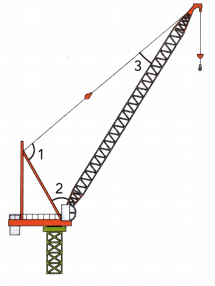# Math in Focus Grade 7 Chapter 6 Review Test Answer Key

Practice the problems of Math in Focus Grade 7 Workbook Answer Key Chapter 6 Review Test to score better marks in the exam.

## Math in Focus Grade 7 Course 2 B Chapter 6 Review Test Answer Key

Concepts and Skills

Tell whether each pair of angles are supplementary, complementary or neither.

Question 1.
m∠1 = 23° and m∠2 = 157°

Explanation: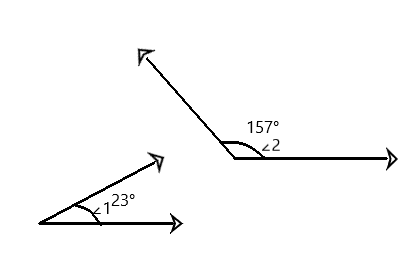Question 2.
m∠3 = 65° and m∠4 = 25°

Explanation: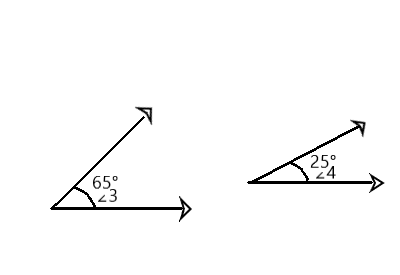Question 3.
m∠5 = 43° and m∠6 = 57°

Explanation: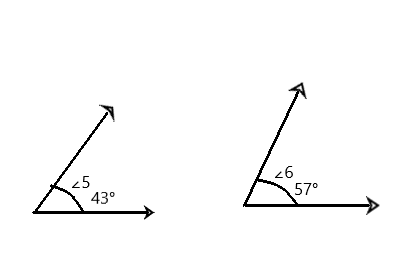Question 4.
m∠7 = 82° and m∠8 = 8°

Explanation: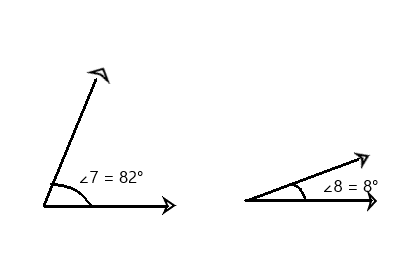Question 5.
m∠9 = 110° and m∠10 = 80°

Explanation: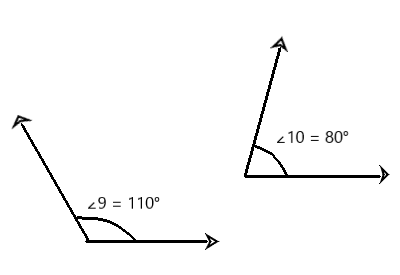Question 6.
m∠11 = 18° and m∠12 = 62°

Explanation: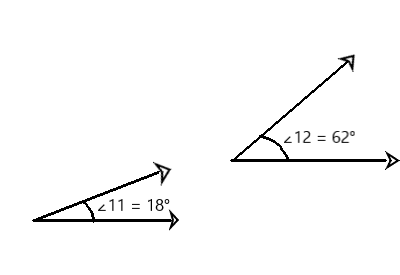Tell whether the following list of angle measures are complementary or supplementary.

m∠A = 67°, m∠B = 80°, m∠C = 131°, m∠D = 21°,
m∠E = 51°, m∠F = 46°, m∠G = 10°, m∠H = 120°,
m∠J = 69°, m∠K = 60°, m∠P = 49°, m∠Q = 113°,
m∠R = 44°, m∠S = 41°

Question 7.
Name two pairs of complementary angles.
m∠B = 80°, m∠G = 10°
m∠F = 46°, m∠R = 44°

Explanation:
Two angles are called complementary when the measures of their angles add to 90 degrees. The two pairs of complementary angles are m∠B = 80°, m∠G = 10°. If we add both angles the sum adds up to 90°.

Question 8.
Name two pairs of supplementary angles.
m∠A = 67°, m∠Q = 113°
m∠H = 120°, m∠K = 60°

Explanation:
Two angles are called supplementary when the measures of their angles add to 180 degrees. The two pairs of supplementary angles are m∠A = 67°, m∠Q = 113°. If we add both angles the sum adds up to 180°.

Copy and complete.

Question 9.
Name two pairs of angles for each type of angle pair.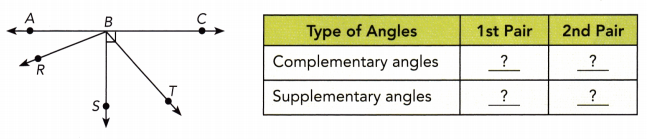∠TBC = 60°, ∠ABR = 30° are complementary angles.
∠RBT = 120°, ∠TBC = 60°are supplementary angles.

Explanation: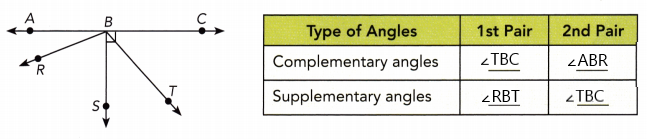The two pairs of angles for complementary angles are ∠TBC = 60°, ∠ABR = 30°.
The two pairs of angles for supplementary angles are ∠RBT = 120°, ∠TBC = 60°

Find the measure of each numbered angle.

Question 10.
$$\overleftrightarrow{\mathrm{AB}}$$ is a straight line.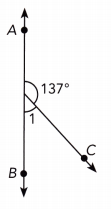The measurement of numbered angle 1 is 43°.

Explanation: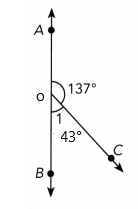∠AOC + ∠BOC = 180°
1 + 137° = 180°
1 = 180° – 137°
1 = 43°

Question 11.
$$\overleftrightarrow{\mathrm{AB}}$$ is a straight line.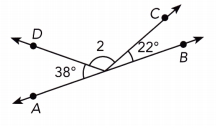The measurement of numbered angle 2 is 120°.

Explanation: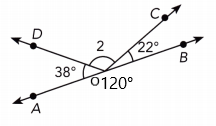∠AOD + ∠DOC + ∠COB = 180°
38° + 2 + 22° = 180°
2 + 60° = 180°
2 = 180° – 60°
2 = 120°

Find the measure of each numbered angle.

Question 12.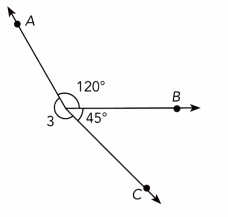The measurement of numbered angle 3 is 195°

Explanation: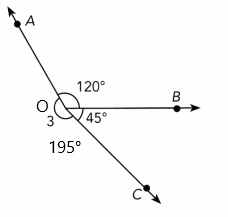∠AOB + ∠COB + ∠AOC = 360°
3 + 120°+ 45° = 360°
3 + 165° = 360°
3 = 360° – 165°
3 = 195°

Question 13.
$$\overleftrightarrow{\mathrm{AB}}$$ is a straight line.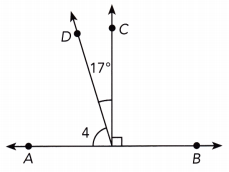The measurement of  numbered angle 4 is 73°

Explanation: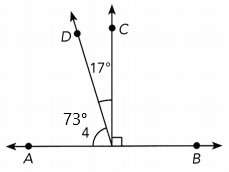∠AOD + ∠DOC + ∠COB = 180°
4 + 17° + 90° = 180°
4 + 107° = 180°
4 = 180° – 107°
4 = 73°

Use an equation to find the value of each variable.

Question 14.
$$\overleftrightarrow{\mathrm{AB}}$$ is a straight line.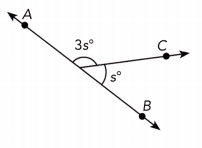s°= 45°, 3s°= 135°

Explanation: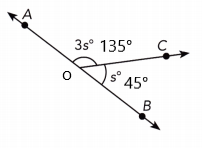∠AOC + ∠COB = 180°
3s° + s° = 180°
4s° = 180°
s° = 180 ÷ 4
s° = 45°
3s°= 135°

Question 15.
$$\overleftrightarrow{\mathrm{AB}}$$ is a straight line.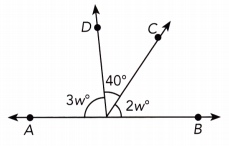w° = 28°, 2w° =56°, 3w°=140°

Explanation: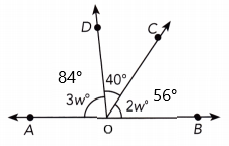∠AOD + ∠DOC + ∠COB = 180°
3w° + 40° + 2w° = 180°
5w° + 40° = 180°
5w° = 180° – 40°
5w° = 140°
w° = 28°
3w°= 3 × 28 = 84°
2w°= 2° × 28 = 56°

Question 16.
$$\overleftrightarrow{\mathrm{AB}}$$ is a straight line.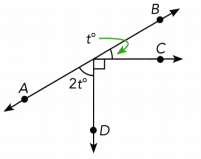t° = 30°, 2t° = 30°

Explanation: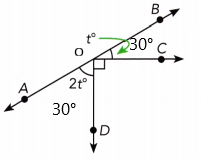∠BOC + ∠AOD + ∠DOC = 180°
t° + 2t° + 90° = 180°
3t° + 90° = 180°
3t° = 180° – 90°
3t° = 90°
t° = 90° ÷ 3
t° = 30°

Question 17.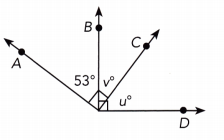v°=17°, u°=73°

Explanation:
∠AOB + ∠BOC = 90°
53° + v° = 90°
v° = 90° – 53°
v° = 17°
∠BOC + ∠COD = 90°
v° + u° = 90°
17° + u° = 90°
u° = 90° – 17°
u° = 73°

Question 18.
$$\overleftrightarrow{\mathrm{AB}}$$, $$\overleftrightarrow{\mathrm{CD}}$$, and $$\overleftrightarrow{\mathrm{EF}}$$ are a straight line.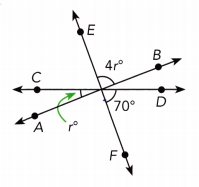r°= 22°, 4r°= 88°

Explanation:
∠COA = ∠BOD = r° (vertically opposite angles)
∠EOB + ∠BOD + ∠FOD = 180°
4r° + r° + 70° = 180°
5r° + 70° = 180°
5r° = 180° – 70°
5r° = 110°
r° = 110 ÷ 5
r° = 22°
4r° = 4 × 22 = 88°

Question 19.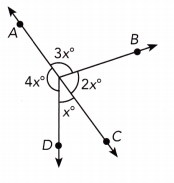x° = 36°, 2x° = 72°, 3x° = 108°, 4x° = 144°

Explanation: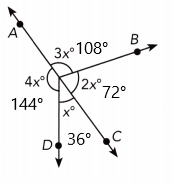3x° + 2x° = 180°
5x° = 180°
x° = 180÷5
x° = 36°
2x° = 36 × 2 = 72°
3x° = 3 × 36 = 108°
4x° = 4 × 36 = 144°

$$\overline{M N}$$ is parallel to $$\overline{P Q}$$. Find the measure of each numbered angle.

Question 20.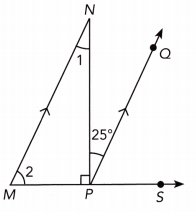∠1 = 25°, ∠2 = 65°, x° = 65°

Explanation:
Let ∠QPS = x°
∠NPM + ∠NPQ + ∠QPS = 180°
90° + 25° + x° = 180°
115° + x° = 180°
x° = 180 -115°
x° = 65°
∠2 = ∠x = 65° Corresponding angles are equal
∠NMP + ∠PNM = 90°
∠2 + ∠1 = 90°
65° + ∠1 = 90°
∠1 = 90° – 65°
∠1 = 25°

Question 21.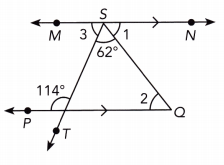∠1 = 52°, ∠2 = 52°, ∠3 = 66°

Explanation:
Let ∠SOQ = x°
114°+ x° = 180°
x° = 180° – 114°
x° = 66°
x° = ∠3 = 66° (vertically opposite angles)
∠3 + ∠TSQ + ∠QSN = 180°
∠3 + 62° + ∠2 = 180°
66° + 62° + ∠2 = 180°
∠2 = 180° – 128°
∠2 = 52°
∠1 = ∠2 (vertically opposite angles)
∠1 = 52°

Problem Solving

Find the value of x.

Question 22.
Find the value of x.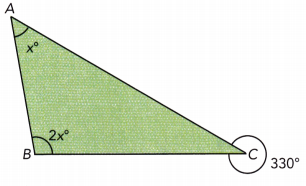x° + 2x° = 330°
3x°= 330°
x° = 330 ÷ 3
x° = 110°

Question 23.
ABCD is a rhombus. Find the measures of ∠1 and ∠2.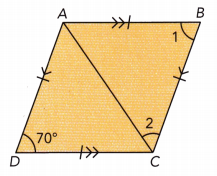∠1 = 70°, ∠2 = 120°

Explanation:
Opposite angles in a rhombus are equal.
∠1 = 70°
∠1 + ∠2 + 70° = 360°
70° + ∠2 + 70° = 360°
∠2 + 140° = 360°
∠2 = 360° – 140°
∠2 = 120°

Question 24.
ABCD is a rectangle. $$\overline{\mathrm{AE}}$$ and $$\overline{\mathrm{DC}}$$ are straight lines. ∠FBG is a right angle, m

ABF = 74°, and m∠BEG = 42°. Find the measures of ∠EBG and ∠BGC.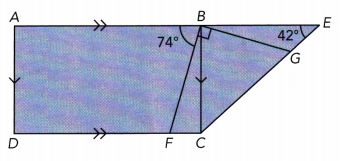x=∠EBG =16°, ∠BGC = 122°
Explanation:
74° + 90° + x° = 180°
x° = 180° – 164°
x° = 16°
16° + 42° + ∠BGC = 180°
∠BGC = 180° – 58°
∠BGC = 122°

Question 25.
The diagram shows the flag of the United Kingdom. m∠MNR = 90°. Name two pairs of complementary and supplementary angles.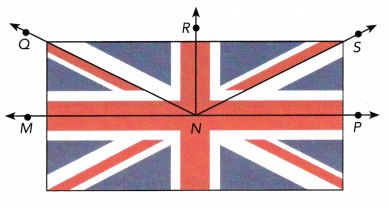∠MNQ, ∠SNP, ∠QNS are complementary angles.
∠MNR, ∠MNQ, ∠SNP are supplementary angles.

Explanation:
The two pairs of angles for complementary angles are ∠MNQ, ∠SNP, ∠QNS.
The two pairs of angles for supplementary angles are ∠MNR, ∠MNQ, ∠SNP.

Question 26.
m∠1 = 15° and m∠2 = 131°. $$\overleftrightarrow{\mathrm{AB}}$$ is a straight line. Find the measure of ∠3.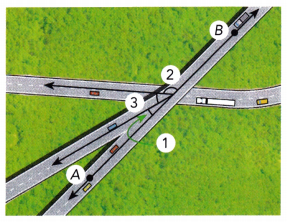∠3 = 34°

Explanation:
m∠1 + m∠2 + m∠3 = 180°
15° + 131° + m∠3 = 180°
m∠3 + 146° = 180°
m∠3 = 180° – 146°
m∠3 = 34°

Question 27.
The diagram shows ∠1 and ∠2, which are formed by $$\overleftrightarrow{M N}$$ intersecting $$\overleftrightarrow{P Q}$$ and $$\overleftrightarrow{R S}$$. In the diagram, m∠1 = (12x + 7)°, m∠2 = (10x + 15)°, and x = 4. Explain how you know that $$\overleftrightarrow{P Q}$$ is parallel to $$\overleftrightarrow{R S}$$.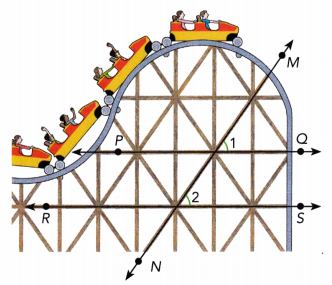Parllel lines are lines that are always the same distance apart and will never intersect. Hence in the given figure the lines $$\overleftrightarrow{P Q}$$ is parallel to $$\overleftrightarrow{R S}$$.
And iif two lines are parllel the corresponding angles are equal. Therefore ∠1 = ∠2

Explanation:
m∠1 = m∠2  are corresponding angles
(12x + 7)° = (10x + 15)°
12x – 10x = 15 – 7
2x = 8
x° = 8 ÷ 2
x° = 4°
(12x + 7)° = 12 × 4 + 7 = 48 + 7 = 55°
(10x +15)° = 10 × 4 + 15 = 40 + 15 = 55°

Question 28.
In the diagram, m∠1 = (5x – 20)°, m∠2 = (2x + 14)°, and m∠3 = 18°. Use an equation to find the measures of ∠1 and ∠2.